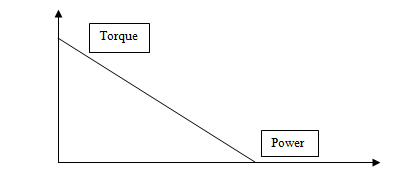# Relation Between torque and Power

## Introduction

Torque refers to an equivalent to the rotational properties in the linear force of the machinery body. Power denotes the work done in a particular duration of time. The inner relation between power and torque in terms of machinery aspects is directly proportional. The power of the machinery particles that are continuously rotating this also denoted as a scholarly product of torque as well as angular velocity. In any kind of rotary motion, measuring the relation between the power and torque of the machinery body is derived from the comparison of the linear equivalent.

## Relation between Torque and Power

The linear displacement of the machinery particles is the actual distance that is covered at any kind of rotational circumference and is given by the angle-covered products as well as the radius. The product that includes linear velocity, as well as time (Andrade et al. 2020), mainly provides linear distances. The linear distance of the machinery body can be measured with the formula Linear distance = radius $\mathrm{\times}$ angular velocity $\mathrm{\times}$ time.

Images Coming soon

The force of the machinery body can be calculated with the formula of Torque/radius and the power is denoted by force $\mathrm{\times}$ liner distance that is divided by the total time taken (Artetxe et al. 2018). Therefore, the relation between the power and torque can be presented as Power = Torque $\mathrm{\times}$ Angular velocity.

## Properties of Torque and Power

Torque can be denoted by the moment of force that is generally measured by the rotation speed of a machinery object that occurred on the axis of the object. As an example, it can be referred to that during the knobbing of a door the torque is commonly used.

It belongs to the vector quantity having magnitude as well as direction. T is the symbol of torque and it is calculated with the formula The T= Frsin ($\mathrm{\theta}$) where F signifies the applied force and r denotes the distance between the point with the pivot of the object, in which force has been applied.

In this formula, $\mathrm{\theta}$ signifies the angle that is made between the applied force and r.

Images Coming soon

The power is the actual rate of the work done that can be measured with the unit of Watt. Watt is named after the inventor of Scottish scientist James Watt. 1 watt is equivalent to the 1-joule of the rate of work done per second (Wiam & Ali, 2019).

In order to measure the power of the machinery body P = duration of E/ duration of T formula is commonly used where P denotes the power of the body, duration of Esignifies the change of the energy of the body and duration of T refers to the time changes during the time.

## Application of Torque and the PowerFlat spiral spring is commonly applied to measure the torque of the machinery body. For measuring the torque in a rotating part of the machinery body different types of machines and a dynamometer are applied. 1 horsepower is equivalent to 746 Watt. The relation between torque and power can be defined as the power being the product of torque as well as the angular velocity (Muduli et al. 2020).

The unit of food-pound can calculate torque and the power of the body can be measured by the watt, which denotes 1 Joule/second.

## Difference between Torque and Power

Torque Power
Torque is defined as the tendency of the force to the rotational objects in a specific direction. The power of the machinery is denoted by the work done rate.
Torque is considered as the vector quantity. The power belongs to the scalar quantity.
It is measured by the newton- meter (N-m) that is the SI unit of Torque. The Watt (W) measures it that is the SI unit of Power.
In order to calculate the torque of the machinery particles The T= Frsin ($\mathrm{\theta}$) formula is also used. In order to calculate the torque of the machinery particles P = duration of E/ duration of T formula is also used.

## Conclusion

Torque is a kind of instantaneous measure that has no type of time component. While power has a type of time component because the RPM refers to the critical function of the power of the different machinery particles. Torque refers to the preliminary phase breaker that is very helpful to pull any kind of vehicle. While the power deals with the moment of the wheel that has been gone out of the static inertia.

## FAQs

Q.1. Does the mass centre of the body essentially lie inside of the body?

Ans. In terms of uniform mass density, the location of the mass centre is equivalent to the geometric centre of the machinery body. The power of the body is calculated by the rate of done work while the torque denotes the rotation force tendency of the machinery particles.

Q.2. What is the measurement process of the power and torque?

Ans. The measurement process of the power and torque of the machinery body can be calculated with the formula of POWER = (TORQUE $\mathrm{\div}$ RADIUS) $\mathrm{\times}$ (RPM $\mathrm{\times}$ RADIUS $\mathrm{\times}$ 2 $\mathrm{\times}$ $\mathrm{\pi}$). The RPM refers to the rotation per minute of the machinery particles.

Q.3. What is the usage of the power and torque that can be seen?

Ans. A practical example of the application of power and torque can be seen in the running of a vehicle. Torque is the preliminary phase breaker while power has a type of time component that can be calculated with the rotation of the wheel per minute.

Q.4. What is the SI unit of power and torque?

Ans. The Si unit is Newton- meter (N-m) of torque. The Watt is the SI unit of the power.